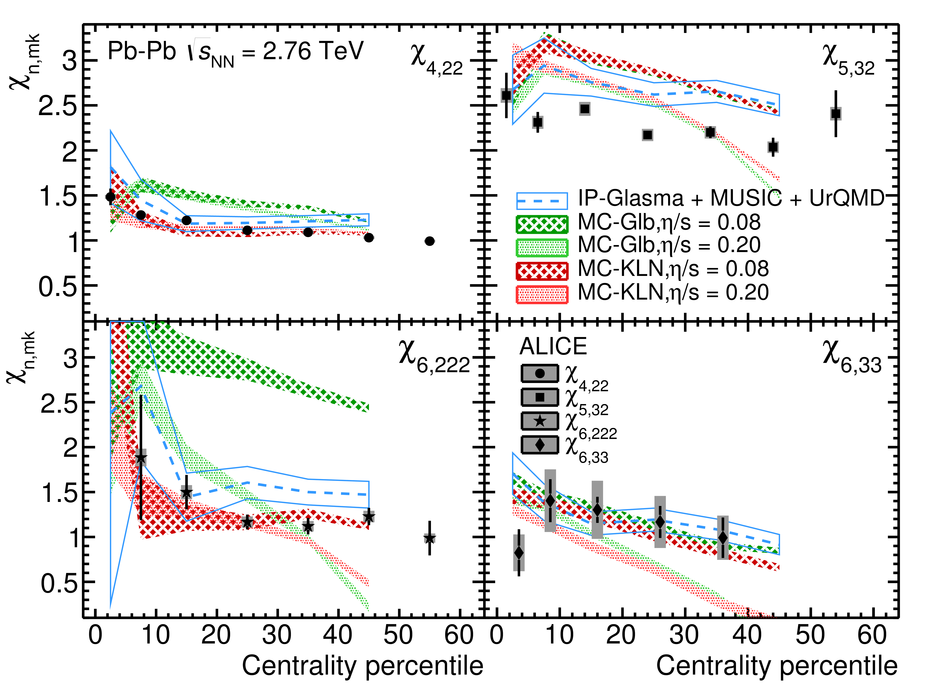# Figure 4

 Centrality dependence of $\chi$ in Pb--Pb collisions at $\sqrt{s_{_{\rm NN}}} = 2.76$ TeV. Hydrodynamic calculations from ${\tt VISH2+1}$  are shown in shaded areas and the one from ${\tt IP}$-${\tt Glasma+MUSIC+UrQMD}$  are shown with open bands.